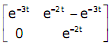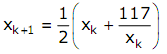# The peak voltage across A and B, with S open is

1.  400/nΠV

2.  800V

3.  4000/ΠV

4.  800/ΠV

4

800/ΠV

Explanation :
No Explanation available for this question

# If the waveform of i(t) is changed to i(t)=10sin(100Πt)A,the peak voltage across A and B with S closed is

1.  400V

2.  240V

3.  320V

4.  160V

4

400V

Explanation :
No Explanation available for this question

# The coils are to be connected to obtain a single phase, 400/1000V, auto transformer to drive a load of 10kVA. Which of the options given should be exercised to realize the required auto transformer

1.  Connect A and D; Common B

2.  Connect A and C; Common B

3.  Connect B and D; Common C

4.  Connect A and C; Common D

4

Connect A and D; Common B

Explanation :
No Explanation available for this question

# In the autotransformer obtained in Question,the current in each coil is

1.  Coil-1 is 25 A and Coil-2 is 10 A

2.  Coil-1 is 10 A and Coil-2 is 25 A

3.  Coil-1 is 10 A and Coil-2 is 15 A

4.  Coil-1 is 15 A and Coil-2 is 10 A

4

Coil-1 is 25 A and Coil-2 is 10 A

Explanation :
No Explanation available for this question

# For the circuit given above,the Thevenin's resistance across the terminals A and B is

1.  0.5kΩ

2.  0.2kΩ

3.  1kΩ

4.  0.11kΩ

4

0.2kΩ

Explanation :
No Explanation available for this question

# For the circuit given above,the Thevenin's voltage across the terminals A and B is

1.  1.25V

2.  0.25V

3.  1V

4.  0.5V

4

0.5V

Explanation :
No Explanation available for this question

# The system transfer function is

1.  s+2/s2+5s-6

2.  s+3/s2+5s+6

3.  s+5/s2+5s+6

4.  2s-5/s2+5s-6

4

s+5/s2+5s+6

Explanation :
No Explanation available for this question

# The state transition matrix of the above system is

1.

2.

3.

4.

4Explanation :
No Explanation available for this question

# It's line integral over the straight line from (x,y)= (0,2) to (x,y) = (2,0) evaluates to

1.  -8

2.  4

3.  8

4.  0

4

0

Explanation :
No Explanation available for this question

# Let x2 -117 = 0.The iterative steps for the solution using Newton-Raphson's method is given by

1.

2.

3.

4.

4